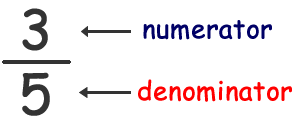# Definition of Numerator and Denominator

### The "top" and "bottom" parts of a fraction...

Here's the simple definition you're probably looking for: The numerator is the top part of a fraction, and the denominator is the bottom part of a fraction.There are plenty of more complicated ways to define a fraction and its numerator and denominator. Remember that a fraction represents a part of a whole. The numerator represents how many parts of that whole are being considered, while the denominator represents the total number of parts created from the whole. In the fraction above, the whole value (say, an apple pie) has been divided into 5 equal parts. If someone has 3/5 of the pie, they have three of those five equal parts.